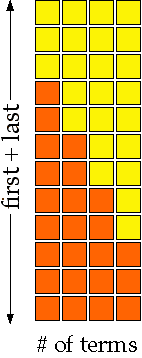# Sequences and Series

Henri PicciottoStaircases: a lesson which can work in different ways and yield different payoffs at various levels: pre-algebra, Algebra 1, Algebra 2, Teachers' Mathematics. I link to it here because it can serve as a preview of arithmetic series. (There are detailed notes and some spoilers on pp. 2-3.)

Lessons from Algebra: Themes, Tools, Concepts by Anita Wah and Henri Picciotto
Arithmetic Sequences and Series | Teacher notes
Geometric Sequences and Series | Teacher notes

Part of the pedagogy behind the above lessons:

From my Math Education Blog:
Geometric in More Than One Way!
An unusual but effective Algebra 2 introduction to sequences:
Iterating Linear Functions
Lessons for 11th and 12th grade about triangular, pentagonal, and Fibonacci numbers:
Stairway to Infinity! Proof by Mathematical Induction
Directory of material on this site that involves integer sequences, with links to the Online Encyclopedia of Integer Sequences:
Integer Sequences